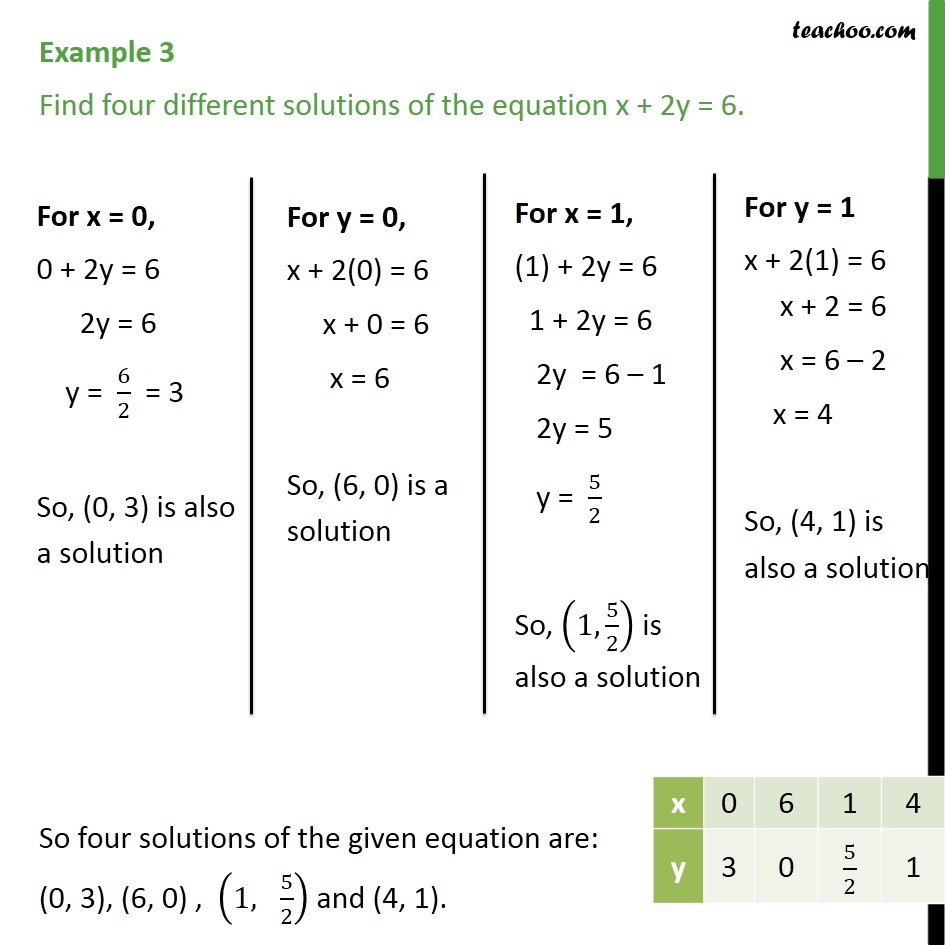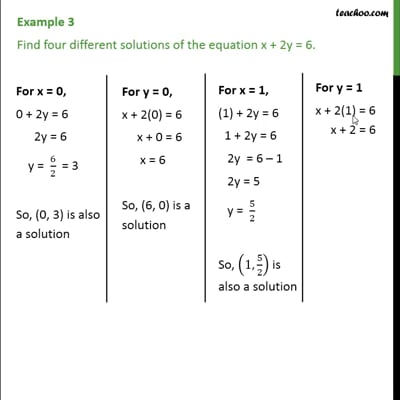Examples

Chapter 4 Class 9 Linear Equations in Two Variables
Serial order wiseThis video is only available for Teachoo black users

Solve all your doubts with Teachoo Black (new monthly pack available now!)

### Transcript

Example 3 Find four different solutions of the equation x + 2y = 6. For x = 0, 0 + 2y = 6 2y = 6 y = 6/2 = 3 So, (0, 3) is also a solution For y = 0, x + 2(0) = 6 x + 0 = 6 x = 6 So, "(6, 0)" is a solution For x = 1, (1) + 2y = 6 1 + 2y = 6 2y = 6 – 1 2y = 5 y = 5/2 So, (1, 5/2) is also a solution For y = 1 x + 2(1) = 6 x + 2 = 6 x = 6 – 2 x = 4 So, (4, 1) is also a solution So four solutions of the given equation are: (0, 3), (6, 0) , (1, 5/2) and (4, 1).